#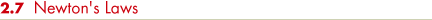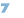Kepler's three laws, which so simplified the solar system, were discovered empirically. In other words, they resulted solely from the analysis of observational data and were not derived from any theory or mathematical model. Indeed, Kepler did not have any appreciation for the physics underlying his laws. Nor did Copernicus understand the basic reasons why his heliocentric model of the solar system worked. Even Galileo, often called the father of modern physics, failed to understand why the planets orbit the Sun.

What prevents the planets from flying off into space or from falling into the Sun? What causes them to revolve about the Sun, apparently endlessly? To be sure, the motions of the planets obey Kepler's three laws, but only by considering something more fundamental than those laws can we really understand these motions. The heliocentric system was secured when, in the seventeenth century, the British mathematician Isaac Newton (Figure 2.17) developed a deeper understanding of the way all objects move and interact with one another as they do.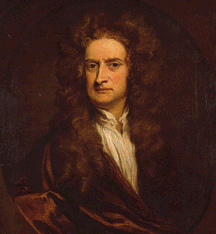Figure 2.17 Sir Isaac Newton (16421727).

### THE LAWS OF MOTION

Isaac Newton was born in Lincolnshire, England, on Christmas Day in 1642, the year that Galileo died. Newton studied at Trinity College of Cambridge University, but when the bubonic plague reached Cambridge in 1665, he returned to the relative safety of his home for 2 years. During that time he made probably the most famous of his discoveries, the law of gravity (although it is but one of the many major scientific advances Newton is responsible for). However, either because he regarded the theory as incomplete or possibly because he was afraid that he would be attacked or plagiarized by his colleagues, he did not tell anyone of his monumental achievement for almost 20 years. It was not until 1684, when Newton was discussing with Edmund Halley (of Halley's comet fame) the leading astronomical problem of the day—Why do the planets move according to Kepler's laws?—that he astounded his companion by revealing that he had solved the problem in its entirety nearly two decades before!

Prompted by Halley, Newton published his theories in perhaps the most influential physics book ever written: Philosophiae Naturalis Principia Mathematica (The Mathematical Principles of Natural Philosophy—what we would today call "science"), usually known simply as Newton's Principia. The ideas expressed in that work form the basis for what is now known as Newtonian mechanics. Three basic laws of motion, the law of gravity, and a little calculus (which Newton also invented) are sufficient to explain and quantify virtually all the complex dynamic behavior we see on Earth and throughout the universe. Newton's laws are listed in More Precisely 2-2.

Figure 2.18 illustrates Newton's first law of motion. The first law simply states that a moving object will move forever in a straight line unless some external force changes its direction of motion. For example, the object might glance off a brick wall or be hit with a baseball bat; in either case, a force changes the original motion of the object. The tendency of an object to keep moving at the same speed and in the same direction unless acted upon by a force is known as inertia. A familiar measure of an object's inertia is its mass—loosely speaking, the total amount of matter it contains. The greater an object's mass, the more inertia it has, and the greater is the force needed to change its state of motion.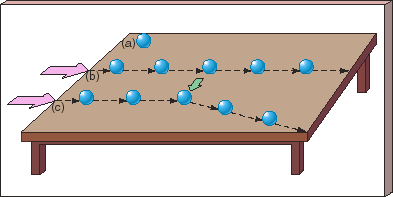Figure 2.18 An object at rest will remain at rest (a) until some force acts on it (b). It will then remain in that state of uniform motion until another force acts on it. The arrow in (c) shows a second force acting at a direction different from the first, which causes the object to change its direction of motion.

Newton's first law contrasts sharply with the view of Aristotle, who maintained (incorrectly) that the natural state of an object was to be at rest—most probably an opinion based on Aristotle's observations of the effect of friction. In our discussion we will neglect friction—the force that slows balls rolling along the ground, blocks sliding across tabletops, and baseballs moving through the air. In any case, it is not an issue for the planets because there is no appreciable friction in outer space. The fallacy in Aristotle's argument was first realized and exposed by Galileo, who conceived of the notion of inertia long before Newton formalized it into a law.

The rate of change of the velocity of an object—speeding up, slowing down, or simply changing direction—is called its acceleration. Newton's second law states that the acceleration of an object is directly proportional to the applied force and inversely proportional to its mass—that is, the greater the force acting on the object, or the smaller the mass of the object, the greater its acceleration. Thus, if two objects are pulled with the same force, the more massive one will accelerate less; if two identical objects are pulled with different forces, the one experiencing the greater force will accelerate more.

Finally, Newton's third law simply tells us that forces cannot occur in isolation—if body A exerts a force on body B, then body B necessarily exerts a force on body A that is equal in magnitude, but oppositely directed.

Only in extreme circumstances do Newton's laws break down, and this fact was not realized until the twentieth century, when Albert Einstein's theories of relativity once again revolutionized our view of the universe (see Chapter 22). Most of the time, however, Newtonian mechanics provides an excellent description of the motion of planets, stars, and galaxies through the cosmos.

### GRAVITY

Forces can act instantaneously or continuously. To a good approximation, the force from a baseball bat that hits a home run can be thought of as being instantaneous in nature. A good example of a continuous force is the one that prevents the baseball from zooming off into space—gravity, the phenomenon that started Newton on the path to the discovery of his laws. Newton hypothesized that any object having mass always exerts an attractive gravitational force on all other massive objects. The more massive an object, the stronger its gravitational pull.

Consider a baseball thrown upward from Earth's surface, as illustrated in Figure 2.19. In accordance with Newton's first law, the downward force of Earth's gravity continuously modifies the baseball's velocity, slowing the initial upward motion and eventually causing the ball to fall back to the ground. Of course, the baseball, having some mass of its own, also exerts a gravitational pull on Earth. By Newton's third law, this force is equal and opposite to the weight of the ball (the force with which Earth attracts it). But, by Newton's second law, Earth has a much greater effect on the light baseball than the baseball has on the much more massive Earth. The ball and Earth feel the same gravitational force, but Earth's acceleration is much smaller.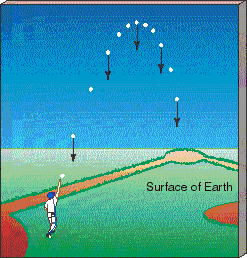Figure 2.19 A ball thrown up from the surface of a massive object such as a planet is pulled continuously by the gravity of that planet (and, conversely, the gravity of the ball continuously pulls the planet).

Now consider the trajectory of the same baseball batted from the surface of the Moon. The pull of gravity is about one-sixth as great on the Moon as on Earth, so the baseball's velocity changes more slowly—a typical home run in a ballpark on Earth would travel nearly half a mile on the Moon. The Moon, less massive than Earth, has less gravitational influence on the baseball. The magnitude of the gravitational force, then, depends on the masses of the attracting bodies. Theoretical insight, as well as delicate laboratory experiments, tells us that the force is in fact directly proportional to the product of the two masses.

Studying the motions of the planets reveals a second aspect of the gravitational force. At locations equidistant from the Sun's center, the gravitational force has the same strength, and it is always directed toward the Sun. Furthermore, detailed calculation of the planets' accelerations as they orbit the Sun reveals that the strength of the Sun's gravitational pull decreases in proportion to the square of the distance from the Sun. (Newton himself is said to have first realized this fact by comparing the accelerations not of the planets but of the Moon and an apple falling to the ground—the basic reasoning is the same in either case.) The force of gravity is said to obey an inverse-square law. As shown in Figure 2.20, inverse-square forces decrease rapidly with distance from their source. For example, tripling the distance makes the force 32 = 9 times weaker, while multiplying the distance by 5 results in a force that is 52 = 25 times weaker. Despite this rapid decrease, the force never quite reaches zero. The gravitational pull of an object having some mass can never be completely extinguished.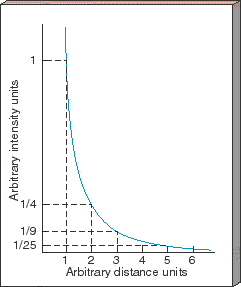Figure 2.20 Inverse-square forces rapidly weaken with distance from their source. The strength of the gravitational force decreases with the square of the distance from the Sun. The force never quite diminishes to zero, however, no matter how far away from the Sun we go.

We can combine the preceding statements about mass and distance to form a law of gravity that dictates the way in which all material objects attract one another. As a proportionality, Newton's law of gravity is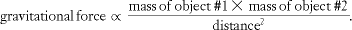(The symbol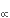here means "is proportional to". See More Precisely 2-2 for a fuller statement of this law.) This relationship is a compact way of stating that the gravitational pull between two objects is directly proportional to the product of their masses and inversely proportional to the square of the distance separating them.

To Newton, gravity was a force that acted at a distance, with no obvious way in which it was actually transmitted from place to place. Newton was not satisfied with this explanation, but he had none better. To appreciate the modern view of gravity, consider any piece of matter having some mass—it could be smaller than an atom or larger than a galaxy. Extending outward from this object in all directions is a gravitational field produced by the matter. We now regard such a field as a property of space itself—a property that determines the influence of one massive object on another. All other matter "feels" the field as a gravitational force.

### PLANETARY MOTION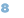The mutual gravitational attraction of the Sun and the planets, as expressed by Newton's law of gravity, is responsible for the observed planetary orbits. As depicted in Figure 2.21, this gravitational force continuously pulls each planet toward the Sun, deflecting its forward motion into a curved orbital path. Because the Sun is much more massive than any of the planets, it dominates the interaction. We might say that the Sun "controls" the planets, not the other way around.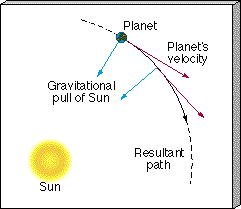Figure 2.21 The Sun's inward pull of gravity on a planet competes with the planet's tendency to continue moving in a straight line. These two effects combine, causing the planet to move smoothly along an intermediate path, which continually "falls around" the Sun. This unending tug-of-war between the Sun's gravity and the planet's inertia results in a stable orbit.

The Sun—planet interaction sketched here is analogous to what occurs when you whirl a rock at the end of a string above your head. The Sun's gravitational field is your hand and the string, and the planet is the rock at the end of that string. The tension in the string provides the force necessary for the rock to move in a circular path. If you were suddenly to release the string—which would be like eliminating the Sun's gravity—the rock would fly away along a tangent to the circle, in accordance with Newton's first law.

In the solar system, at this very moment, Earth is moving under the combined influence of these two effects: the competition between gravity and inertia. The net result is a stable orbit, despite our continuous rapid motion through space. In fact, Earth orbits the Sun at a speed of about 30 km/s, or some 70,000 mph. (Verify this for yourself by calculating how fast Earth must move to complete a circle of radius 1 A.U.—and hence of circumference 2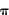A.U., or 940 million km—in 1 year, or 3.2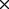107 seconds. The answer is 9.4108 km/ 3.1107s, or 30.3 km/s.) More Precisely 2-3 describes how astronomers can use Newtonian mechanics and the law of gravity to measure the masses of Earth, the Sun, and many other astronomical objects by studying the orbits of objects near them.

### KEPLER'S LAWS RECONSIDERED

Newton's laws of motion and the law of universal gravitation provided a theoretical explanation for Kepler's empirical laws of planetary motion. Just as Kepler modified Copernicus's model by introducing ellipses rather than circles, so too did Newton make corrections to Kepler's first and third laws. It turns out that a planet does not orbit the exact center of the Sun. Instead, both the planet and the Sun orbit their common center of mass. Because the Sun and the planet feel equal and opposite gravitational forces (by Newton's third law), the Sun must also move (by Newton's first law), driven by the gravitational influence of the planet. The Sun is so much more massive than any planet that the center of mass of the planet—Sun system is very close to the center of the Sun, which is why Kepler's laws are so accurate. Thus, Kepler's first law becomes

The orbit of a planet around the Sun is an ellipse, with the center of mass of the planet—Sun system at one focus.

As shown in Figure 2.22, however, the center of mass for two objects of comparable mass does not lie within either object. For identical masses (Figure 2.22a), the orbits are identical ellipses, with a common focus located midway between the two objects. For unequal masses (as in Figure 2.22b), the elliptical orbits still share a focus and both have the same eccentricity, but the more massive object moves more slowly and on a tighter orbit. (Note that Kepler's second law, as stated earlier, continues to apply without modification to each orbit separately, but the rates at which the two orbits sweep out area are different.) In the extreme case of a planet orbiting the much more massive Sun (Figure 2.22c), the path traced out by the Sun's center lies entirely within the Sun itself.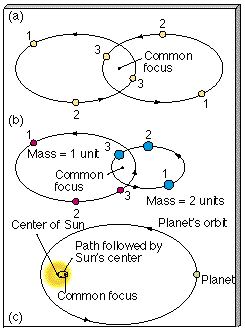Figure 2.22 (a) The orbits of two bodies (stars, for example) with equal masses, under the influence of their mutual gravity, are identical ellipses with a common focus. That focus is not at the center of either star but instead is located at the center of mass of the pair, midway between them. The positions of the two bodies at three different times are indicated by the pairs of numbers. (Notice that a line joining the bodies always passes through the common focus.) (b) The orbits of two bodies, one of which is twice as massive as the other. Again, the elliptical orbits have a common focus, and the two ellipses have the same eccentricity. However, in accordance with Newton's laws of motion, the more massive body moves more slowly, and in a smaller orbit, staying closer to the center of mass (at the common focus). In this particular case, the larger ellipse is twice the size of the smaller one. (c) In this extreme case of a hypothetical planet orbiting the Sun, the common focus of the two orbits lies inside the Sun.

The change to Kepler's third law is also small in the case of a planet orbiting the Sun but very important in other circumstances, such as the orbital motion of two stars that are gravitationally bound to each other. Following through the mathematics of Newton's theory, we find that the true relationship between the semi-major axis a (measured in astronomical units) of the planet's orbit relative to the Sun and its orbital period P (in Earth years) is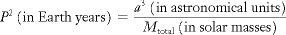where M total is the combined mass of the two objects. Notice that Newton's restatement of Kepler's third law preserves the proportionality between P2and a3, but now the proportionality includes Mtotal, so it is not quite the same for all the planets. The Sun's mass is so great, however, that the differences in Mtotal among the various combinations of the Sun and the other planets are almost unnoticeable, and so Kepler's third law, as originally stated, is a very good approximation. This modified form of Kepler's third law is true in all circumstances, inside or outside the solar system.

### ESCAPING FOREVER

The law of gravity that describes the orbits of planets around the Sun applies equally well to natural moons and artificial satellites orbiting any planet. All our Earth-orbiting, human-made satellites move along paths governed by a combination of the inward pull of Earth's gravity and the forward motion gained during the rocket launch. If the rocket initially imparts enough speed to the satellite, it can go into orbit. Satellites not given enough speed at launch (such as intercontinental ballistic missiles, ICBMs) fail to achieve orbit and fall back to Earth (Figure 2.23). (Technically, ICBMs actually do orbit Earth's attracting center, but their orbits intersect Earth's surface.)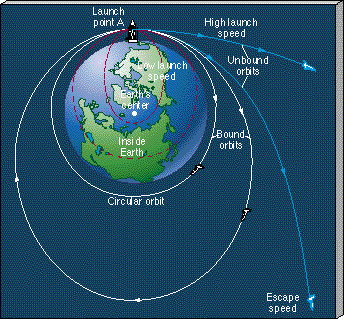Figure 2.23 The effect of launch speed on the trajectory of a satellite. With too low a speed at point A the satellite will simply fall back to Earth. Given enough speed, however, the satellite will go into orbit it "falls around Earth." As the initial speed at point A is increased, the orbit will become more and more elongated. When the initial speed exceeds the escape speed, the satellite will become unbound from Earth and will escape along a hyperbolic trajectory.

Some space vehicles, such as the robot probes that visit the other planets, attain enough speed to escape our planet's gravitational field and move away from Earth forever. This speed, known as the escape speed, is about 41 percent greater (actually, (check)2 = 1.414...times greater) than the speed of a circular orbit at any given radius.*

*In terms of the formula presented earlier (see More Precisely 2-3), the escape speed is given by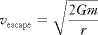.

At less than escape speed, the adage "what goes up must come down" (or at least stay in orbit) still applies. At more than the escape speed, however, a spacecraft will leave Earth for good (neglecting the effect of air resistance on its way through Earth's atmosphere and assuming that we don't turn the craft around using an on-board rocket motor). Planets, stars, galaxies—all gravitating bodies—have escape speeds. No matter how massive the body, gravity decreases with distance. As a result, the escape speed diminishes with increasing separation. The farther we go from Earth (or any gravitating body), the easier it becomes to escape.

The speed of a satellite in a circular orbit just above Earth's atmosphere is 7.9 km/s (roughly 18,000 mph). The satellite would have to travel at 11.2 km/s (about 25,000 mph) to escape from Earth altogether. If an object exceeds the escape speed, its motion is said to be unbound, and the orbit is no longer an ellipse. In fact, the path of the spacecraft relative to Earth is a related geometric figure called a hyperbola. If we simply change the word ellipse to hyperbola, the modified version of Kepler's first law still applies, as does Kepler's second law. (Kepler's third law does not extend to unbound orbits because it doesn't make sense to talk about a period in those cases.)

Newton's laws explain the paths of objects moving at any point in space near any gravitating body. These laws provide a firm physical and mathematical foundation for Copernicus's heliocentric model of the solar system and for Kepler's laws of planetary motions. But they do much more than that. Newtonian gravitation governs not only the planets, moons, and satellites in their elliptical orbits but also the stars and galaxies in their motion throughout our universe—as well as apples falling to the ground.